# 22 calculus questions 1

1.

Use your graphing calculator to evaluate. (2 points)

 0 π e3 1

2.(2 points)

 does not exist 1 −1 0

3.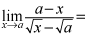(2 points)2a

4.

Find. (2 points)

 Does not exist 4 3 0

5.

Ifand, then find. (2 points)

 64 -4 16 28

6.

Evaluate. (2 points)

 0 1 -1 does not exist

7.

Evaluate. (2 points)

 164

8.

Evaluate. (2 points)

 1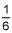Does not exist

9.

If f is a continuous function with odd symmetry and, which of the following statements must be true? (2 points)

I.II. There are no vertical asymptotes.

III. The lines y = 6 and y = -6 are horizontal asymptotes

 All statements are true. I only II only III only

10.

What are the horizontal asymptotes of the function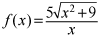? (2 points)

 y = 5 only y = -5 only y = -5 and y = 5 y = 0

11.

Which one or ones of the following statements is/are true? (2 points)

I. If the line y = 2 is a horizontal asymptote of y = f(x), then f is not defined at y = 2.

II. If f(5) > 0 and f(6) < 0, then there exists a number c between 5 and 6 such that f(c) = 0.

III. If f is continuous at 2 and f(2)=8 and f(4)=3, then.

 All statements are true. I only II only III only

12.

Find(2 points)

 0 4 -∞ ∞

13.

Evaluate. (2 points)

 -∞ ∞ 0 2

14.

Which of the following are the equations of all horizontal and vertical asymptotes for the graph of? (2 points)

 y = 1, x = -3, x = 3 y = 0, x = -3, x = 0, x = 3 y = 1, x = -3, x = 0, x = 3 y = 0, x = -3, x = 3

15.

Evaluate. (2 points)

 does not exist 3 8 1

16.

Where isdiscontinuous? (2 points)

 x = -2 x = 4 x = -2 and x = 4 f(x) is continuous everywhere

17.

Which of the following are continuous for all real values of x? (2 points)

I.II.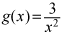III.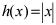I only II only I and II only I and III only

18.

Which of the following must be true for the graph of the function? (2 points)

There is:

I. a vertical asymptote at x = 3

II. a removable discontinuity at x = 3

III. an infinite discontinuity at x = 3

 I only II only III only I, II, and III

19.

What is the average rate of change of y with respect to x over the interval [1, 5] for the function y = 4x + 2? (2 points)

 82 4

20.

What is the instantaneous slope of y =at x = 3? (2 points)21.

The height, s, of a ball thrown straight down with initial speed 32 ft/sec from a cliff 128 feet high is s(t) = -16t2 – 32t + 128, where t is the time elapsed that the ball is in the air. What is the instantaneous velocity of the ball when it hits the ground? (2 points)

 -96 ft/sec 256 ft/sec 0 ft/sec 112 ft/sec

22.

The surface area of a right circular cylinder of height 4 feet and radius r feet is given by S(r)=2πrh+2πr2. Find the instantaneous rate of change of the surface area with respect to the radius, r, when r = 4. (2 points)

 24π 16π 64π 20π